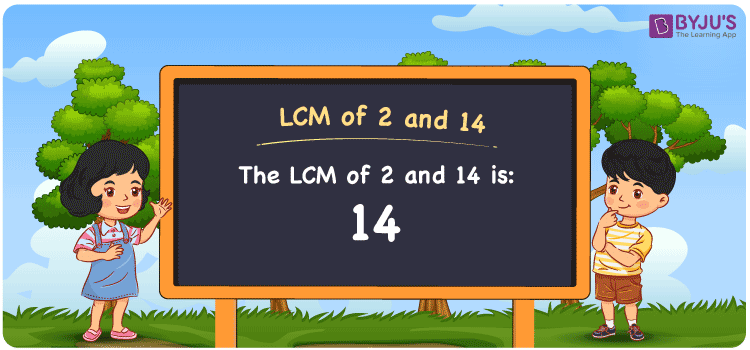# LCM of 2 and 14

LCM of 2 and 14 is 14. The smallest number among all common multiples of 2 and 14 is the LCM of 2 and 14. (2, 4, 6, 8, 10, etc.) and (14, 28, 42, 56, 70, etc.) are the first few multiples of 2 and 14, correspondingly. To find the LCM of 2 and 14, there are basically three methods: prime factorization, listing multiples, and division. In Maths, the LCM of any two numbers is the value which is evenly divisible by the given two numbers.

Also read: Least common multiple

## What is LCM of 2 and 14?

The answer to this question is 14. The LCM of 2 and 14 using various methods is shown in this article for your reference. The LCM of two non-zero integers, 2 and 14, is the smallest positive integer 14 which is divisible by both 2 and 14 with no remainder.## How to Find LCM of 2 and 14?

LCM of 2 and 14 can be found using three methods:

• Prime Factorisation
• Division method
• Listing the multiples

### LCM of 2 and 14 Using Prime Factorisation Method

The prime factorisation of 2 and 14, respectively, is given by:

(2) = 21 and

14 = (2 × 7) = 21 × 71

LCM (2, 14) = 14

### LCM of 2 and 14 Using Division Method

We’ll divide the numbers (2, 14) by their prime factors to get the LCM of 2 and 14 using the division method (preferably common). The LCM of 2 and 14 is calculated by multiplying these divisors.

 2 2 14 7 1 7 x 1 1

No further division can be done.

Hence, LCM (2, 14) = 14

### LCM of 2 and 14 Using Listing the Multiples

To calculate the LCM of 2 and 14 by listing out the common multiples, list the multiples as shown below

 Multiples of 2 Multiples of 14 2 14 4 28 6 42 8 56 10 70 12 84 14 98

The smallest common multiple of 2 and 14 is 14.

Therefore LCM (2, 14) = 14

## Video Lesson on Applications of LCM## LCM of 2 and 14 Solved Example

The product of two numbers is 28. If their GCD is 2, what is their LCM?

Solution:

Given: GCD = 2

product of numbers = 28

∵ LCM × GCD = product of numbers

⇒ LCM = Product/GCD = 28/2

Therefore, the LCM is 14.

The probable combination for the given case is LCM(2, 14) = 14.

## Frequently Asked Questions on LCM of 2 and 14

### What is the LCM of 2 and 14?

The LCM of 2 and 14 is 14. To find the LCM (least common multiple) of 2 and 14, we need to find the multiples of 2 and 14 (multiples of 2 = 2, 4, 6, 8 . . . . 14; multiples of 14 = 14, 28, 42, 56) and choose the smallest multiple that is exactly divisible by 2 and 14, i.e., 14.

### List the methods used to find the LCM of 2 and 14.

The methods used to find the LCM of 2 and 14 are Prime Factorization Method, Division Method and Listing multiples.

### Which of the following is the LCM of 2 and 14? 45, 36, 25, 14

The value of LCM of 2, 14 is the smallest common multiple of 2 and 14. The number satisfying the given condition is 14.

### What is the Relation Between GCF and LCM of 2, 14?

The following equation can be used to express the relation between GCF and LCM of 2 and 14, i.e. GCF × LCM = 2 × 14.

### If the LCM of 14 and 2 is 14, Find its GCF.

LCM(14, 2) × GCF(14, 2) = 14 × 2
Since the LCM of 14 and 2 = 14
⇒ 14 × GCF(14, 2) = 28
Therefore, the GCF (greatest common factor) = 28/14 = 2.